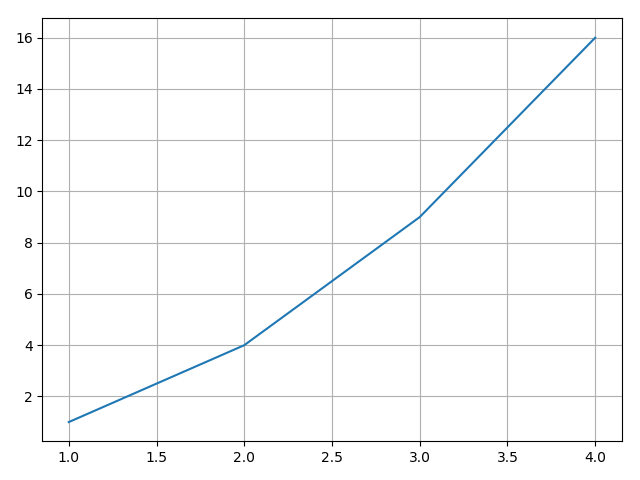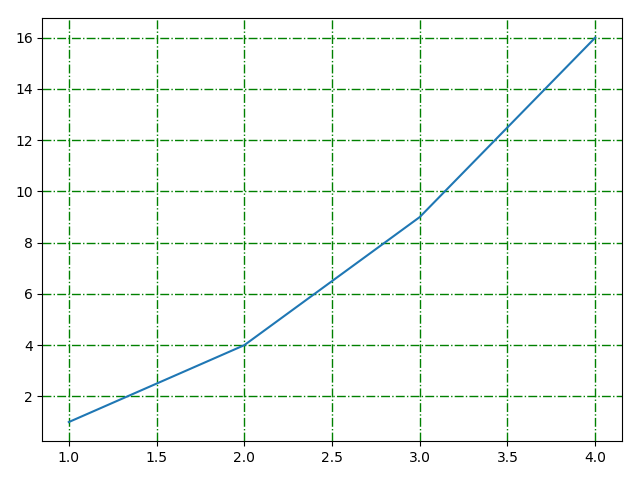# Matplotlib可视化（十三）–网格587-王同学

, ,

## 在背景添加网格

• plt方法

import numpy as np
import matplotlib.pyplot as plt
y = np.arange(1, 5)
plt.plot(y, y**2)
plt.grid(True)#生成网格
# 交互式，可以改变网格颜色、取消网格等等
plt.show()• 面向对象法

import numpy as np
import matplotlib.pyplot as plt
y = np.arange(1, 5)
fig = plt.figure()
plt.plot(y, y**2)
ax.grid(True, color='g', linestyle='-.', linewidth=1)
plt.show()### 评论 抢沙发### 觉得文章有用就打赏一下文章作者

#### 支付宝扫一扫打赏#### 微信扫一扫打赏Vieu3.3主题Q Q 登 录